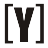# Simple Interest Calculator

This calculator is very useful for calculating the amount of interest and total amount easily and quickly.

how to use this calculator first choose a currency, if your currency does not exist, choose others. Enter the value of the principal amount, annual interest rate, time period (days / weeks / months / quarters / yearly) then push the calculate button to get the calculated amount of interest and total amount.

 Select currency: Dollar (\$) Pound (£) Euro (€) Krona (kr) Leu (L) Lira (t) Peso (P) Ringgit (RM) Rupee (R) Rupee (Rs) Shekel (₪) Zloty (zl) - other - Principal amount: \$ Annual interest rate: % per year Period: Days Weeks Months Quarters Years Interest amount: \$ Total amount: \$

## How to Calculate Simple Interest?

Simple Interest Amount Formula
 = principal amount x rate x n m

simple interest amount is equal to the principal amount multiply by annual interest rate divided by (m) the number of periods per year, multiplied by (n) the number of periods.

Example:
1. If principal amount of \$20.000, annual interest rate of 12% and time of 24 months. Calculate Interest amount and total amount?

Solution:

 = \$ 20.000 x 12 % x 24 months 12 months/year
 Interest Amount = \$ 4800
 Total Amount = \$ 24.800YoosFuhl.com Would you like to receive notifications on latest updates? No Yes